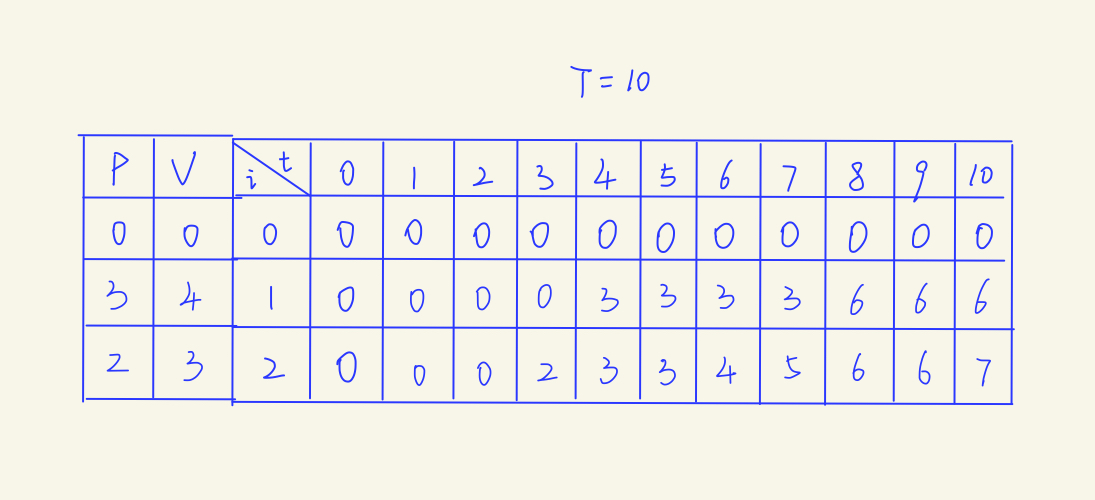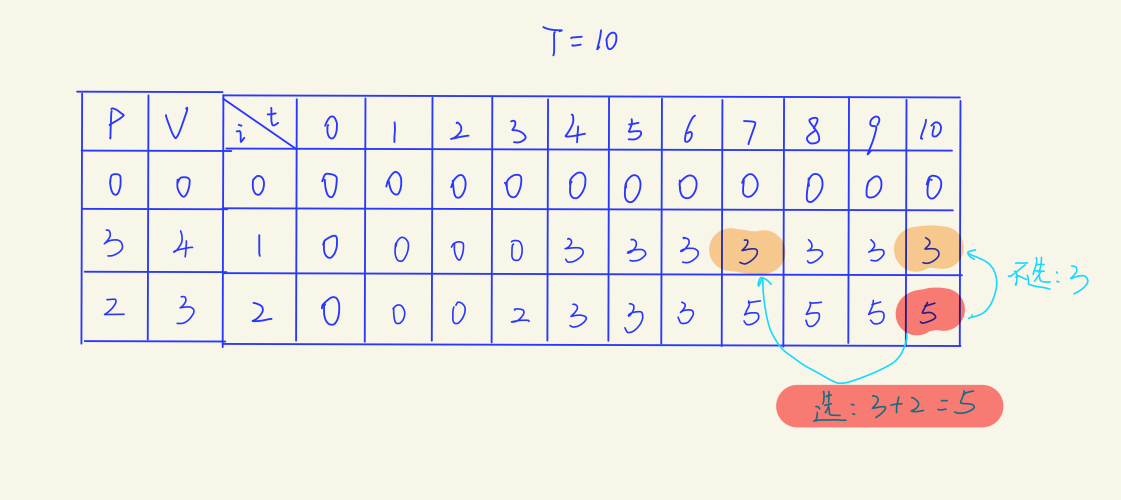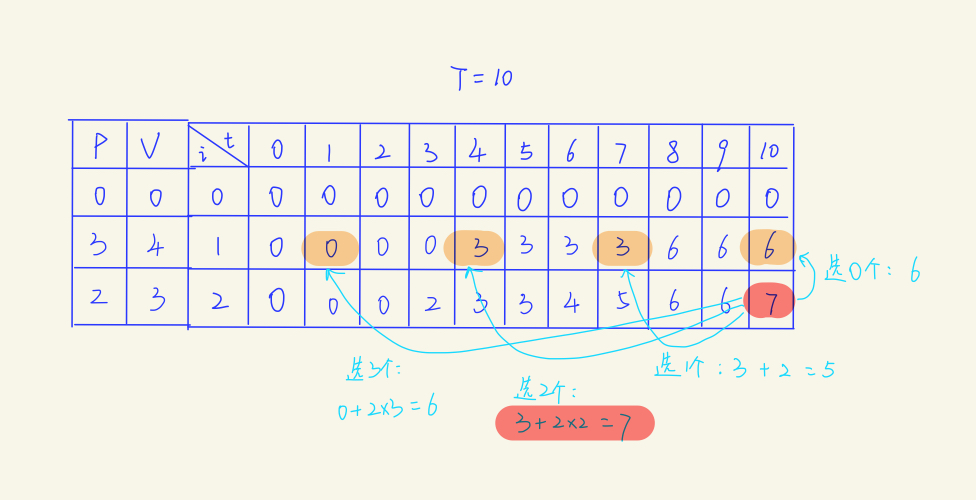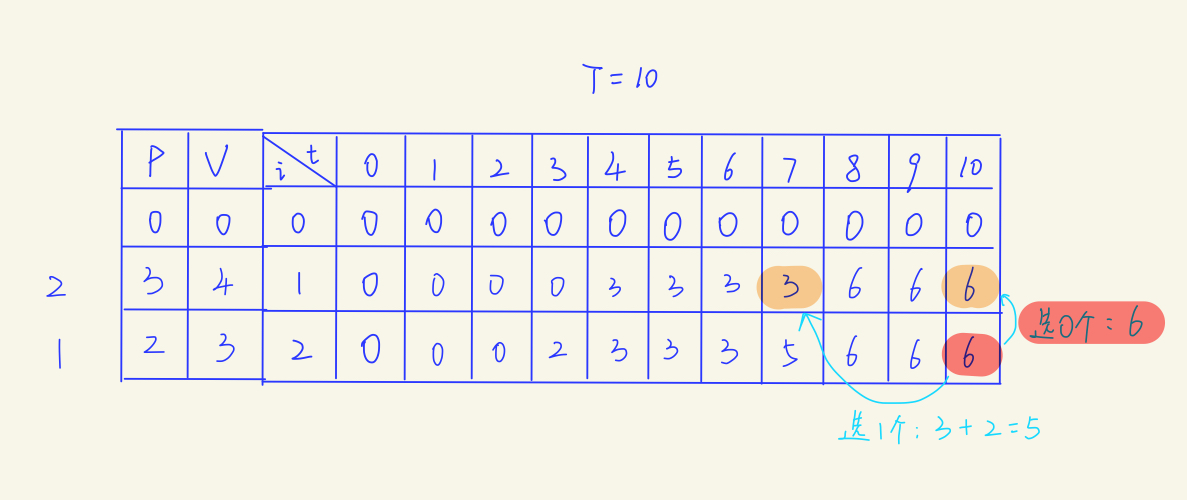# 【动态规划】一次搞定三种背包问题

【动态规划】01背包问题

【动态规划】01背包问题【续】

【动态规划】完全背包问题

【动态规划】多重背包问题

## 三种背包问题的比较

01背包：

01背包的状态转移方程：

F[i,v] = max{F[i-1,v], F[i-1,v-Ci] + Wi}


F[i,v] = max{F[i-1,v-kCi] + kWi | 0 <= kCi <= v}


F[i,v] = max{F[i-1,v-kCi] + kWi | 0 <= k <= Mi}


01背包考虑的是选和不选，所有只需要比较两种策略的最大值即可，而完全背包和多重背包要考虑的是选几个的问题。01背包的填表法：01背包：

for i <- 1 to N
for v <- V to Ci
F[v] = max{F[v],F[v-Ci] + Wi}


def ZeroOneKnapsack(F,C,W)
for v <- V to C
F[v] = max{F[v],F[v-C] + W}


for i <- 1 to N
ZeroOneKnapsack(F,Ci,Wi)


N代表物品数量，Ci代表第i个物品占用的容量，V代表背包总容量，Wi代表第i个物品的价值，下同。

for i <- 1 to N
for v <- Ci to V
F[v] = max{F[v],F[v-Ci] + Wi}


def CompleteKnapsack(F,C,W)
for v <- C to V
F[v] = max{F[v],F[v-C] + W}


for i <- 1 to N
CompleteKnapsack(F,Ci,Wi)


for i <- 1 to N
if v < Ci * Mi
F[v] = max{F[v],F[v-Ci] + Wi}
else
for v <- Ci to V
k <- 1
while k < M && v > Ci * k
F[v] = max{F[v],F[v-Ci*k] + Wi*k}
k++


def MultiKnapsack(F,C,W,M)
if C * M >= V
CompleteKnapsack(F,C,W)
return
else
k <- 1
while k < M
ZeroOneKnapsack(F,KC,KW)
k++
return


for i <- 1 to N
MultiKnapsack(F,Ci,Wi,Mi)


Mi 代表第i件物品最多可选数量

## 混合背包问题

for i <- 1 to N
if 第i件物品属于01背包
ZeroOneKnapsack(F,Ci,Wi)
else if 第i件物品属于完全背包
CompleteKnapsack(F,Ci,Wi)
else if 第i件物品属于多重背包
MultiKnapsack(F,Ci,Wi,Mi)


## 总结posted @ 2019-05-11 18:17  弗兰克的猫  阅读(38519)  评论(7编辑  收藏  举报